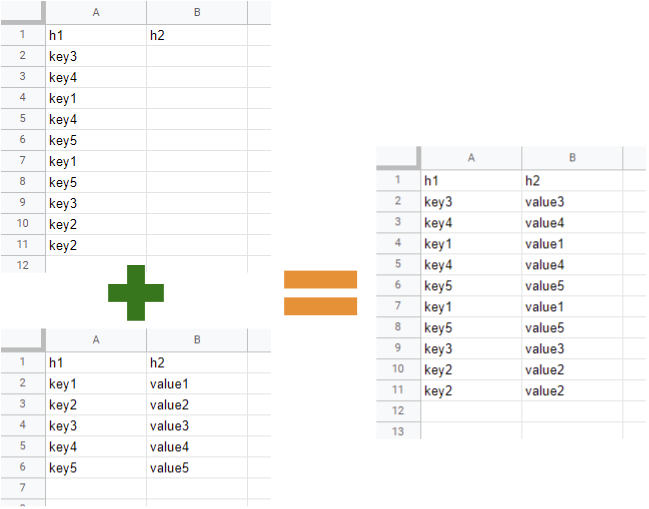# Updating Values of Sheet A with Values of Sheet B using Google Apps Script

GistsThis is a sample script for updating the values of “Sheet A” with the values of “Sheet B” using Google Apps Script. I often see this situation at Stackoverflow and other sites. So, in this post, I would like to introduce the sample script using Google Apps Script.

## Sample script

function myFunction() {
const [src, dst] = ["Sheet1", "Sheet2"].map((e) => ss.getSheetByName(e));
const obj = src
.getRange("A2:B" + src.getLastRow())
.getValues()
.reduce((o, [a, b]) => ((o[a] = b), o), {});
const values = dst
.getRange("A2:A" + dst.getLastRow())
.getValues()
.map(([b]) => [obj[b] || ""]);
dst.getRange(2, 2, values.length, 1).setValues(values);
}


Of course, this situation can be also achieved with the built-in formula of Spreadsheet. For example, when the above image is used, the same result with the column “B” can be obtained at the column “C” by putting a formula of =ARRAYFORMULA(VLOOKUP(A2:A11,Sheet2!A2:B6,2)) to the cell “C2”.